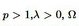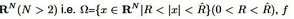##### Nonexistence of Radial Positive Solutions for A Quasilinear Elliptic Equations nonpositone Problems in an Annulus

Weihui Wang, Zuodong Yang

Journal of Advances in Mathematics and Computer Science, Page 1-11
DOI: 10.9734/BJMCS/2013/1767

In this paper, our main purpose is studying the nonexistence of radial positive solutions for the
boundary-value problem:whereis an annulus inis a continuous nonlinear function and satisﬁes f(0) < 0 (the nonpositone case), f also has more than one zero.

##### Distributions of Sum, Difference, Product and Quotient of Independent Non-central Beta Type 3 Variables

Daya K. Nagar, Yeison Arley Ramirez-Vanegas

Journal of Advances in Mathematics and Computer Science, Page 12-23
DOI: 10.9734/BJMCS/2013/1895

Let X and Y be independent random variables, X having a gamma distribution with shape parameter a and Y having a non-central gamma distribution with shape and non-centrality parameters b and δ , respectively. Define Z = X(X+ 2Y ). Then, the random variable Z has a non-central beta type 3 distribution, Z ∼ NCB3(a, b; δ). In this article we derive density functions of sum, difference, product and quotient of two independent random variables each having noncentral beta type 3 distribution. These density functions are expressed in series involving first hypergeometric function of Appell.

##### An Iterative Method for Approximating the Common Solutions of Nonexpansive Semigroups, a System of Variational Inequalities, Variational Inequalities and Equilibrium Problems

Meng Wen, Changsong Hu

Journal of Advances in Mathematics and Computer Science, Page 24-43
DOI: 10.9734/BJMCS/2013/1598

In this work, we consider an general iterative method for finding a common element of the set of common fixed points of a one-parameter nonexpansive semigroup problem, a system of variational inequality problems, a variational inequality problem and the set of solutions of a suitable equilibrium problem in a real Hilbert space. Further we establish a strong convergence theorem based on this method. Our results improve and extend corresponding ones announced by many others.

##### Banach Space X-Valued Bilateral Sequence Space I ( z, x, y, p)

Riti Agrawal, J. K. Srivastava

Journal of Advances in Mathematics and Computer Science, Page 44-51
DOI: 10.9734/BJMCS/2013/2162

Aims/ objectives:
In this paper we introduce and study vector–valued bilateral sequence spacewe investigate the conditions connected with the comparison of the classes in terms ofso that a class is contained in or equal to another class of same kind. We also study topological linear structure of this space when this space is topolized by a suitable paranorm.

##### Recovering Sturm–Liouville Operators on a Graph from Pairwise Disjoint Spectra

I. Dehghani, A. Jodayree Akbarfam

Journal of Advances in Mathematics and Computer Science, Page 52-72
DOI: 10.9734/BJMCS/2013/1991

In this paper, we study an inverse spectral problem for the Sturm-Liouville equation on a three-star graph with the Neumann and Dirichlet boundary conditions in the boundary vertices and matching conditions in the internal vertex. As spectral characteristics, we consider the spectrum of the main problem together with the spectra of two Neumann-Dirichlet problems and one Dirichlet-Dirichlet problem on the edges of the graph and investigate their properties and asymptotic behavior. We prove that if these four spectra do not intersect, then the inverse problem of recovering the potential is uniquely solvable . We give an algorithm for the construction of the potential corresponding to this quadruple of spectra.

##### Asymptotic Expressions of Several Distributions on the Sphere

C.K. Li

Journal of Advances in Mathematics and Computer Science, Page 73-85
DOI: 10.9734/BJMCS/2013/1798How to define the products of distributions is a difficult and not completely understood problem, and has been studied from several points of views since Schwartz established the theory of distributions by treating singular functions as linear and continuous functions on the testing function space. Many fields, such as differential equations or quantum mechanics, require such multiplications. In this paper, we use the Temple delta sequence and the convolution given on the regular manifolds to derive an invariant theorem, that powerfully changes the products of distributions of several dimensional spaces into the well-defined products of a single variable. With the help of the invariant theorem, we solve a couple of particular distributional products and hence we are able to obtain asymptotic expressions for $$\displaystyle \delta^{(k)}(\frac{1}{a(r)}(r - t))$$ as well as the distribution $$\displaystyle \delta^{(k)}(\frac{1}{a(r)}(r^2 - t^2))$$ by the Fourier transform, where the distribution $$\delta^{(k)}(r - t)$$ focused on the sphere $$O_t$$ is defined by $(\delta^{(k)}(r - t), \, \phi) = \frac{(-1)^k}{t^{n - 1}} \int_{O_t} \frac{\partial ^k}{\partial r^k }(\phi r^{n - 1}) d O_t.$